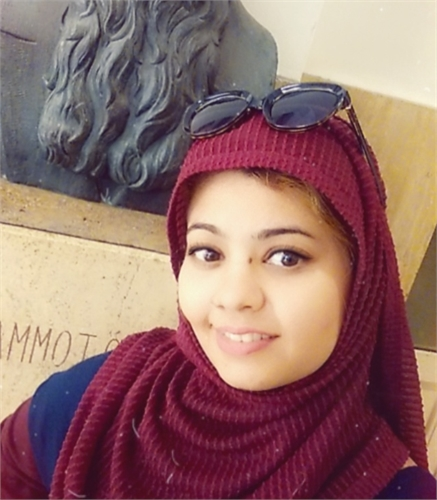## AFSHEEN AYUB

Dottoressaciclo: XXXIII

relatore: Micol Amar

Titolo della tesi: Homogenization of Heat Conduction with Imperfect Interface Involving Laplace-Beltrami operator

We study the asymptotic behavior, as $\varepsilon$ tends to zero, of two independent heat conduction problems with imperfect inclusions. The non-standard problems are set in a finely mixed composite medium consisting of two constituents that are periodically distributed and separated by thermally active interfaces. The novelty of the work is the assumptions on thermal potentials that are coupled by means of interface conditions governed by the Laplace-Beltrami Operator. For the first problem $\PAepsi$, the flux of the thermal potential is assumed to be continuous across the interface, and the jump of the thermal potential is described in terms of the Laplace-Beltrami operator where the source term is the outer flux of the thermal potential. For the second problem $\PBepsi$, the flux of the potential is not continuous and is assumed proportional to the Laplace-Beltrami operator of the sum of the solution $\uepsi$. Here, the jump of the solution $\uepsi$ is governed by an equation involving the Laplace-Beltrami operator and the source is the sum of the outer and inner flux of the thermal potential $\uepsi$. The macroscopic behavior of the material and specifically the overall conductivity is formally and rigorously investigated via the two-scale expansion method and the Periodic unfolding method, respectively. We investigated the homogenized limit for different values of scaling parameter $m \in \{-1, 0, 1\}$, which appears on the interface. These models are then compared in terms of physical meanings and mathematical issues with each other and are available in the literature.

Produzione scientifica

• 11573/1306955 - 2019 - Cancer diagnosis using deep learning: A bibliographic review (01g Articolo di rassegna (Review))
MUNIR, KHUSHBOO; ELAHI, HASSAN; AYUB, AFSHEEN; FREZZA, FABRIZIO; RIZZI, ANTONELLO
• 11573/1562592 - 2021 - Frequency analysis of functionally graded bi-layered cylindrical shells with ring support by galerkin technique (01a Articolo in rivista)
AYUB, AFSHEEN

© Università degli Studi di Roma "La Sapienza" - Piazzale Aldo Moro 5, 00185 Roma